# D6 projected to 3D using the E8 to H4 Folding Matrix

This blogs.ams.org/visualinsight article is an American Mathematical Society post based on some of my work. I’ve made an animation of the D6 polytope with 60 vertices and 480 edges of 6D length Sqrt(2). It is similar to the one in the article by Greg Egan. This version was made from my VisibLie E8 demonstration software.

Best viewed in HD mode.

The process for making this using the .nb version of VisibLie E8 ToE demonstration:

• slide “inches” to 4 (lowers the px resolution)
• select “3D”
• set bckGrnd “b” (black)
• uncheck the shwAxes box
• check the physics box (to give it interesting vertex shapes/colors)
• change the fileExt to “.avi” and check the fileOut box (to save the .avi)
• check the artPrint box (to give it cylinder-like edges)

2) Go to the #9) HMI nD Human Interface pane:

• set pScale to .08 (to increase the vertex shape sizes)

2b) Inside the pane selections:

• set “eRadius” to .01 (for thicker edge lines)

3) Go to the #2) Dynkin pane and interactively create (or select from the drop down menu) the D6 diagram.
BTW – this actually selects the subset of vertices from the E8 polytope that are in D6.

4) Go to the #3) E8 Lie Algebra pane:

• E8->H4 projection
• check the edges box
• “spin” animation
• 30 animation “steps”

or simply upload this file or copy / paste the following into a file with extension of “.m” and select it from the “input file” button on the #9 HMI nD Human Interface pane.

(* This is an auto generated list from e8Flyer.nb *)
new :={
artPrint=True;
inches=4;
physics=True;
p3D=” 3D”;
bckGrnd=GrayLevel;
fileExt=”.avi”;
shwAxes=False;
scale=0.08;
selPrj=”E8->H4″;
lie=”D6″;
cylR=0.01;
showEdges=True;
steps=30;
pthRot=”Spin”;
selPrj=”E8->H4″;
p3D=” 3D”;
};new;

In the next version I will just make this another “MetaFavorite”, so it will be in the dropdown menu in all versions.
Enjoy!# Fun with 8D rotations of E8

This is a little clip containing three different 8 dimensional rotations of E8. While it may seem like a simple rotation of an object in 3-space, if you look carefully (especially the first rotation), you can see sets of vertices moving in different directions.

The object (the 240 vertices of E8 along with 1220 of 6720 edges of 8D length Sqrt) is not “moving”. It is the camera that is moving in an 8 dimensional space.

Best when viewed in HD!

The previous 3D animations here and here are created by projecting the 8 dimensional object of E8 into 3 and simply rotating (spinning) that object around in 3-space. I like to call those animations 4D, since animation visualizes changes over time – a 4D space-time object if you will…

# E8 to 3D projection using my H4 folding matrix

Best viewed in HD mode…

Last year I showed that the Dynkin diagram of the Lie Algebra, Group and Lattice of E8 is related to the Coxeter-Dynkin of the 600 Cell of H4 through a folding of their diagrams: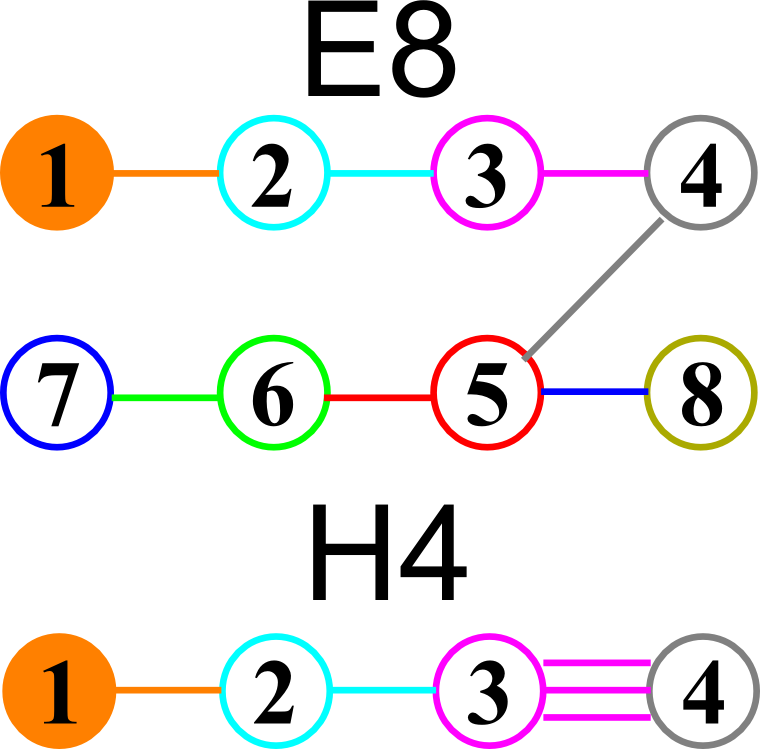I had found the E8 to H4 folding matrix several years ago after reading several papers by Koca and another paper by Dechant, on the topic. The matrix I found is: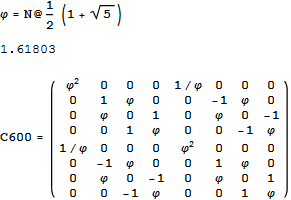Notice that C600=Transpose[C600] and the QuaternionOctonion like structure (ala. Cayley-Dickson) within the folding matrix. Only the first 4 rows are needed for folding, but I use the 8×8 matrix to rotate 8D vectors easily.

The following x,y,z vectors project E8 to its Petrie projection on one face (or 2 of 6 cubic faces, which are the same).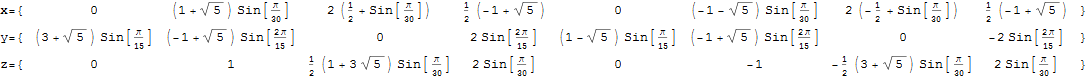On another face are the orthonormal H4 600 cell and the concentric H4/Phi. There are 6720 edges with 8D Norm’d length of Sqrt, but I only show 1220 in order to prevent obscuring the nodes.

These values were calculated using the dot product of the Inverse[4*C600] with the following H4 Petrie projection vertices (aka. the Van Oss projection), where I’ve added the z vector for the proper 3D projection. Notice I am still using 8D basis vectors with the last 4 zero, as this maintains scaling due to the left-right symmetries in C600).The Split Real Even (SRE) E8 vertices are generated from the root system for the E8 Dynkin diagram and its resulting Cartan matrix: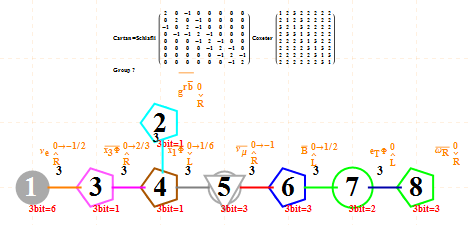This is input from my Mathematica “VisibLie” application. It is shown with the assigned physics particles that make up the simple roots matrix entries: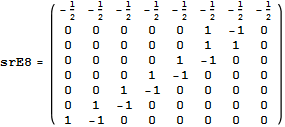.
Please note, the Cartan matrix can also be generated by srE8.Transpose[srE8]

It takes Transpose[srE8] and applies the dot product against the 120 positive and 120 negative algebra roots generated by the Mathematica “SuperLie” package (shown along with its Hasse diagram, which I generate in the full version of my Mathematica notebook):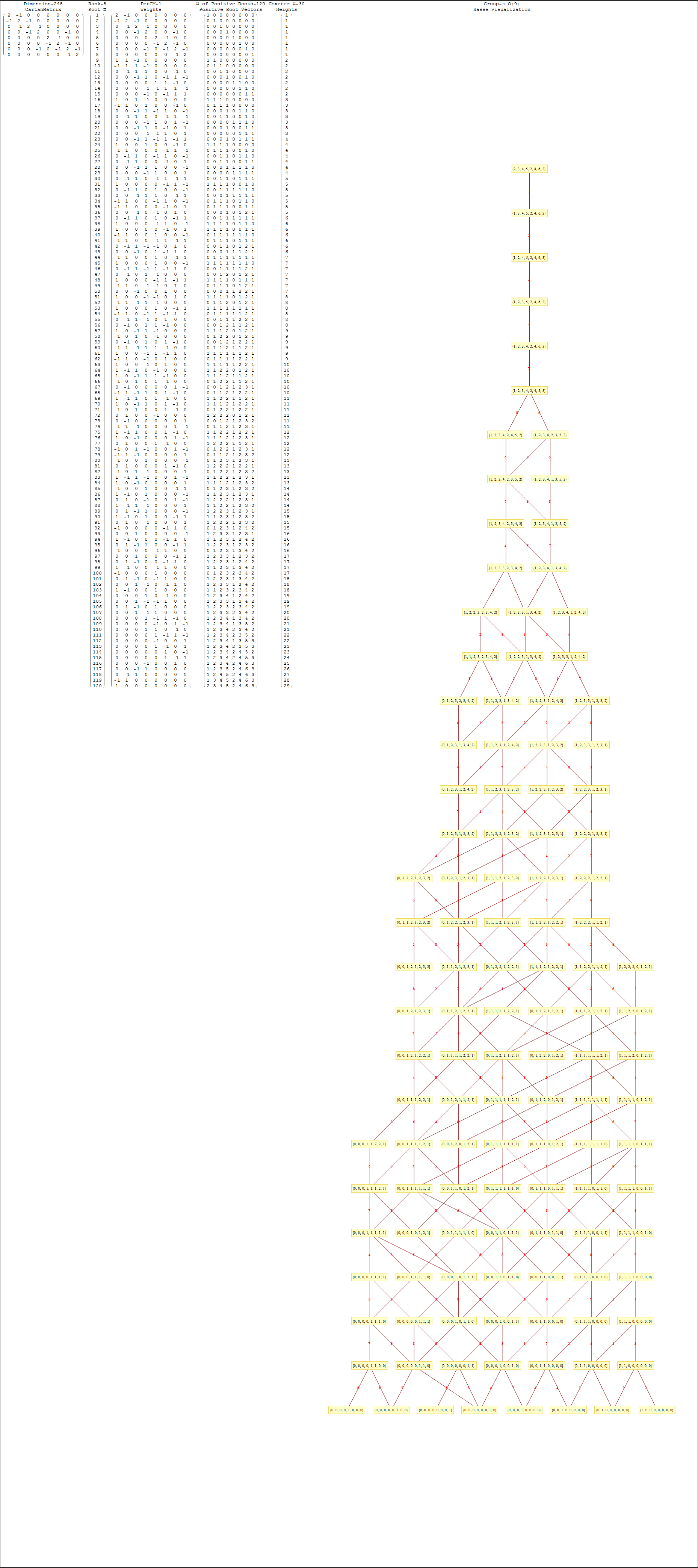Interestingly, E8 in addition to containing the 8D structures D8 and BC8 and the 4D Polychora, contains the 7D E7, as well as the 6D structures of the Hexeract or 6 Cube. I also showed several years ago that this projects down to the 3D as the Rhombic Triacontahedron. The Rhombic Triacontahedron (of Quasicrystal fame) contains the Platonic Solids including the Icosahedron and Dodecahedron! This is shown to be done through folding of the 6-Cube using rows 2 through 4 of the E8 to H4 folding matrix!!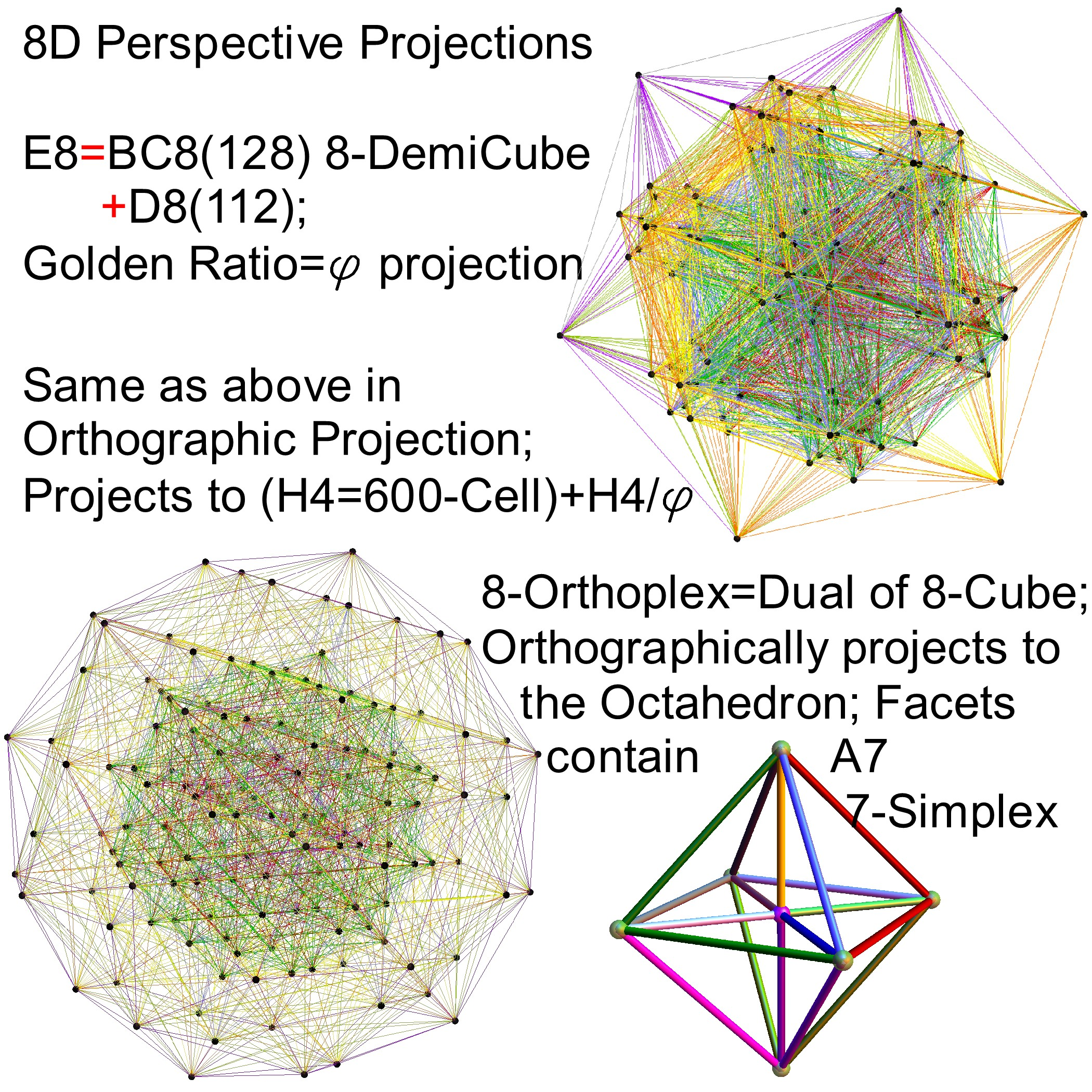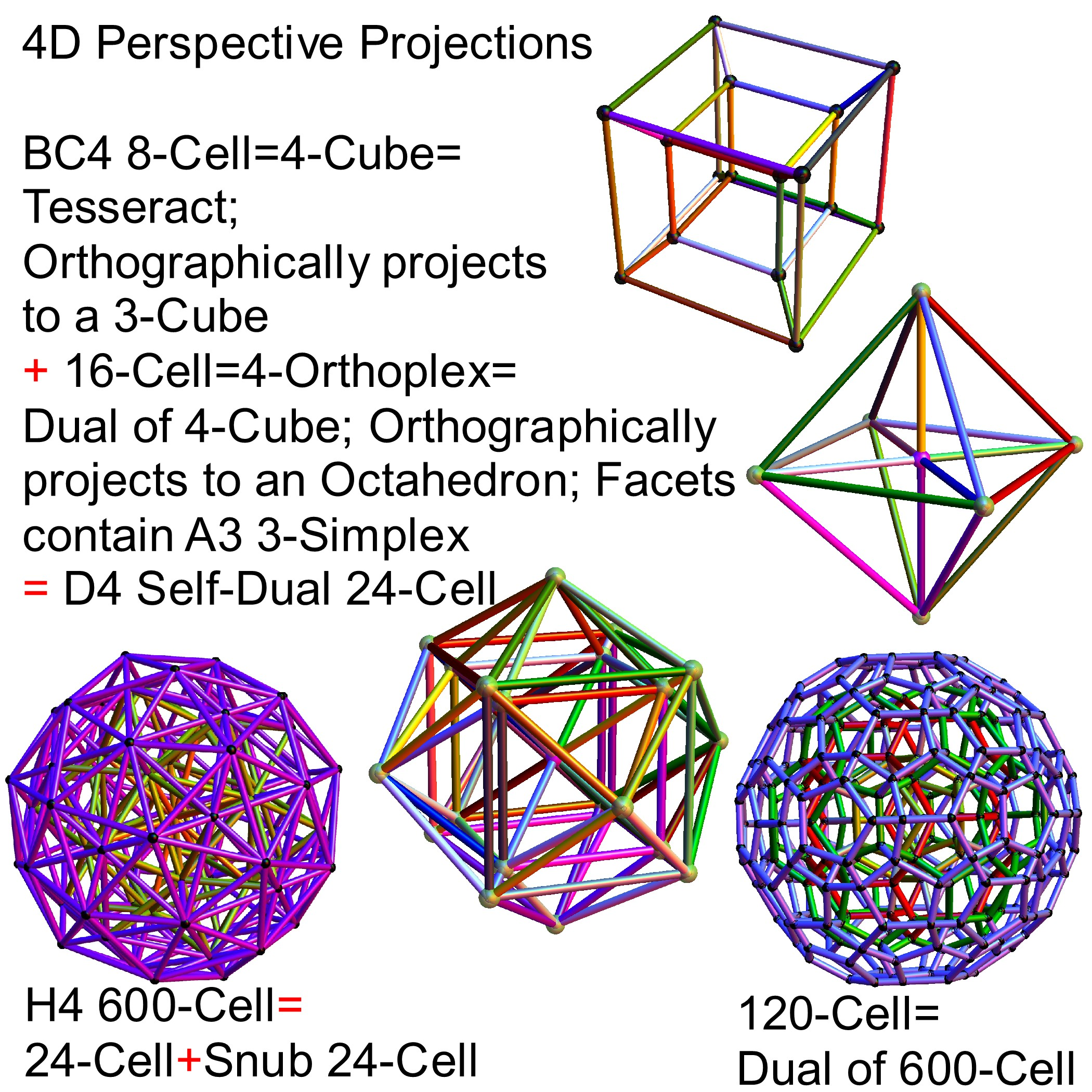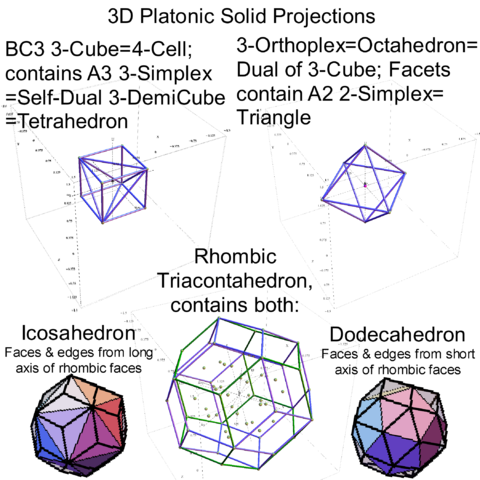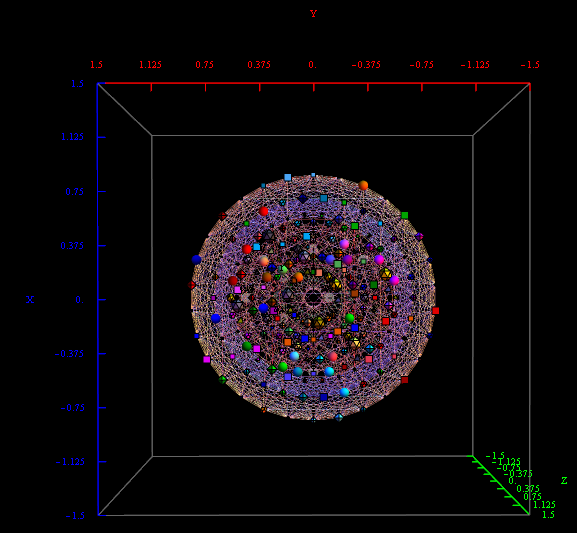# New TheoryOfEverything Visualizer Features

I cleaned up some of the N-Body physics screens, and created a few animation sequences showing the simulation runs available (if you have Mathematica and source code (available upon request and appropriate use-case)).

This is a video of a preliminary Galaxy formation in N-Body gravitational physics.

This is a video of the solar system (not yet using the OpenCL N-Body code for GPU parallelism).

This is a video of the Compton Effect in 3D, which I plan on using to show how Big Bang Inflationary Quantum effects are explained.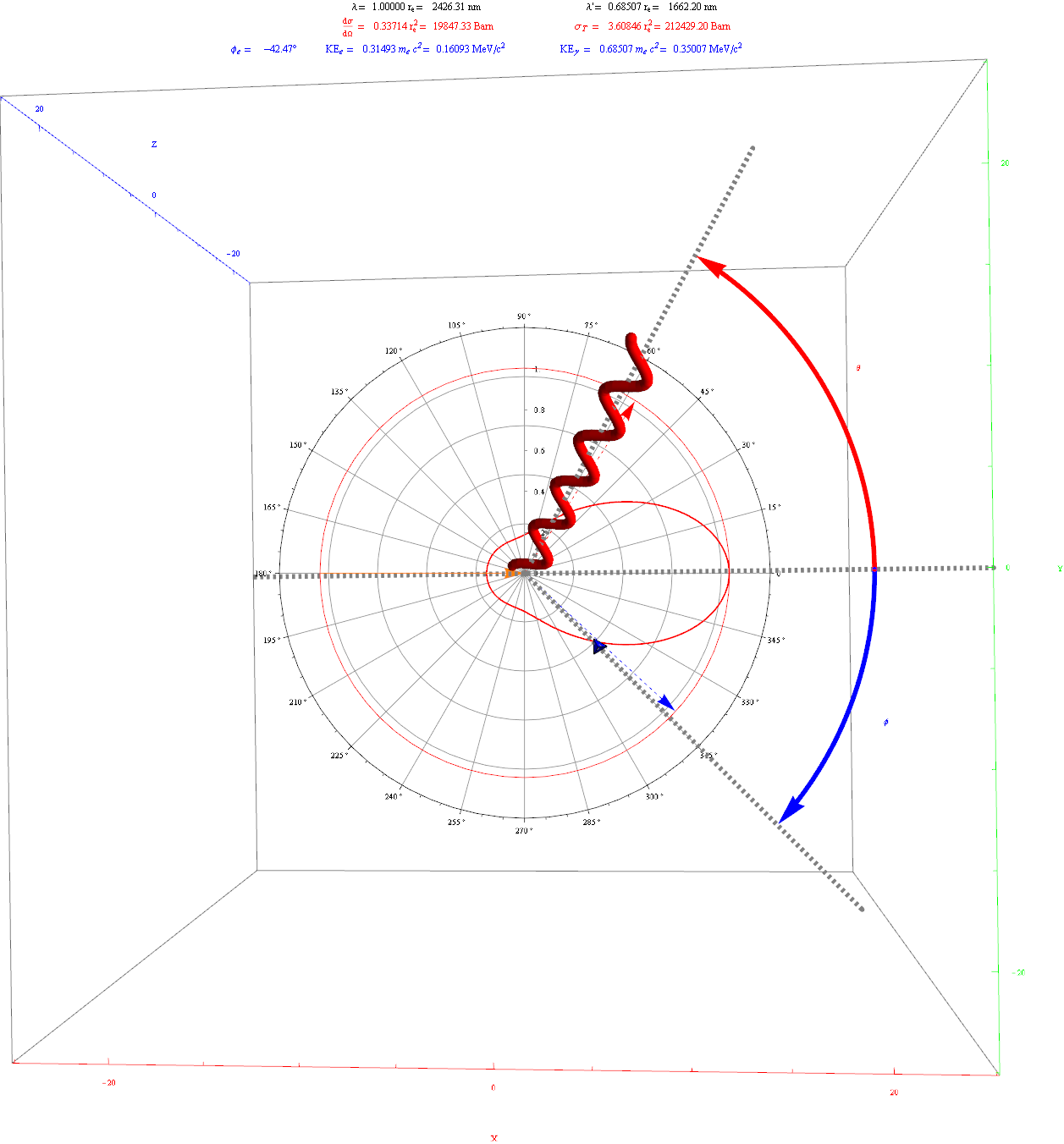I’ve also improved the capabilities of the other demonstrations.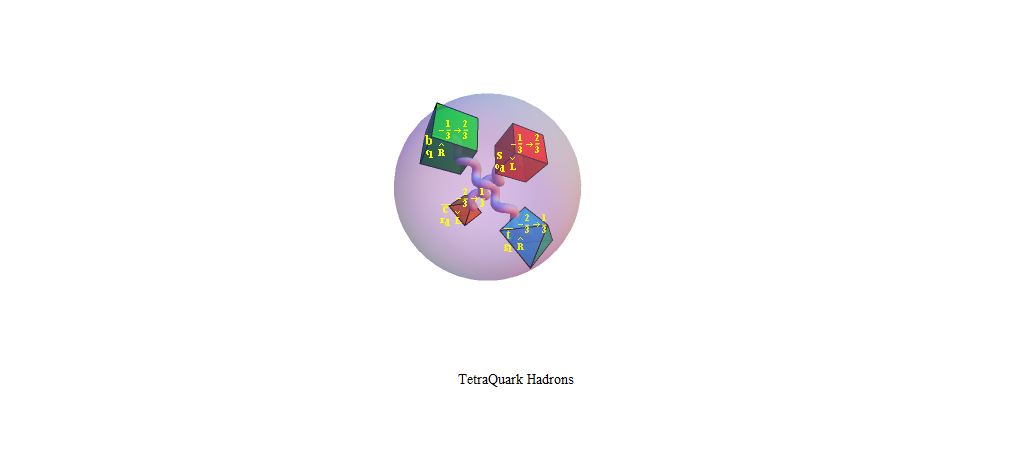# E8 to 4D (3D+T)

E8 8D to 4D (3D+T) Animation

Shown in this animation are the 240 vertices of E8 with shape, size, and color assigned based on theoretical physics of an extended Standard Model (eSM). It is made up of three sets of 120 frames, each with a different algorithm for calculating perspective and orthogonal, rotational and translational 8D flight paths. It is interesting to note that it is the 8D camera that is moving through 8D space and the vertices remain in their same 8D position.

The 30 blue triangles represent E8 triality relationships using an 8D rotation matrix based on 2Pi/3 (or 120 degrees). Each vertex in a blue triangle is transformed into an adjacent one by the dot product with the matrix. A second transformation transforms it to the next, while the third recovers the original vertex.

The 28 red and green triangles are created from a subset of the 6720 (shortest) edges of 8D norm’d length Sqrt(2). These are filtered to represent the particle sums (linked by a red line) for a common (clicked) vertex (linked by 2 green lines). It is interesting to note that all sums for a given vertex are only found in adjacent vertices.

Higher definition (2 sets 60 frames each):

# The 3rd (Z) basis vector for Bathsheba & Wizzy’s 600 Cell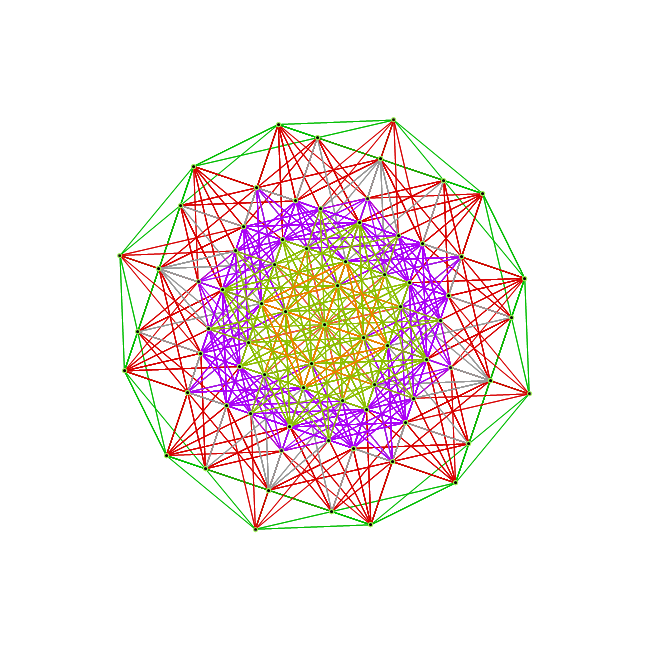While the 3D model I used to create the 2D Van Oss projection isomorphic to E8 Petrie (and a beautiful pentagonal view), it was not the same as what was being used by Richter in his 3D “pre-Van-Oss” construction. Given my H (or x) and V (or y), the 3rd basis vector for this projections is most likely:
Z={0, -0.0801064, 0, 0.236818, 0, 0.0801064, 0, -0.236818}
which reproduces the Richter, Bathsheba and Wizzy’s 3D models. Interestingly, it produces one face (shown above) that is the same as all the orthonormal faces of 2 concentric 600 Cells (at the Golden Ratio). The 3rd unique face is: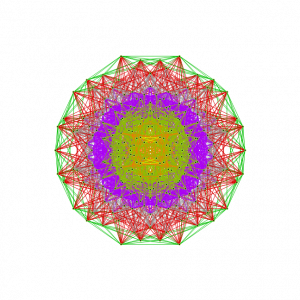I replaced the 3D spin movie of this on my main page with this new projection.